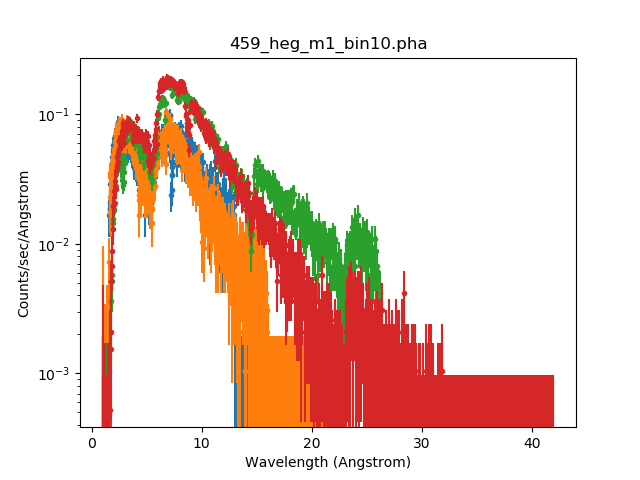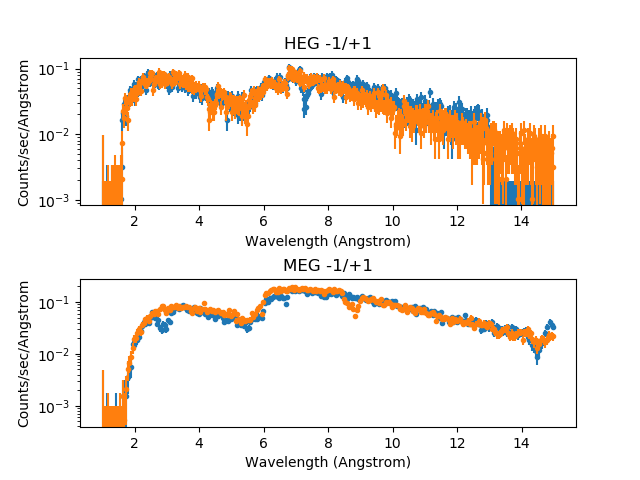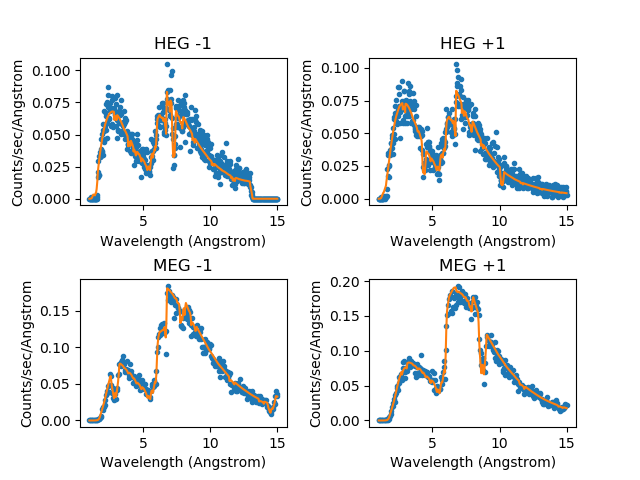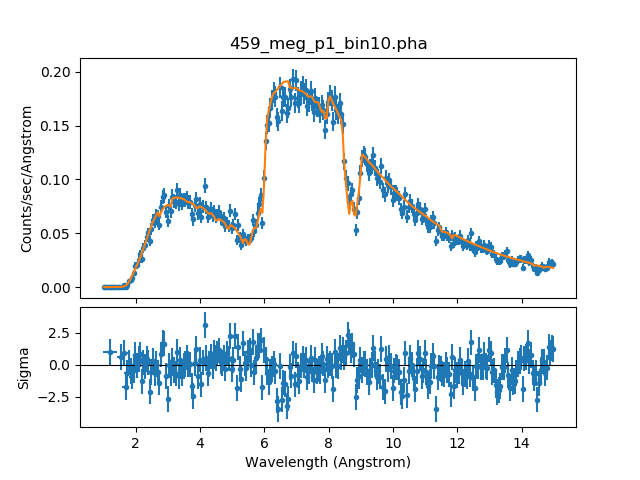# Simulating Chandra ACIS-S LETG Spectra with Sherpa: Establishing the Source Model Expression## Overview

#### Synopsis:

The thread "Simulating Chandra ACIS-S LETG Spectra with Sherpa" illustrates the use of the Sherpa fake_pha command to simulate a spectrum of a point source with the ACIS-S/LETG detector/grating configuration aboard Chandra, using a source model tailored to an existing ACIS-S/HETG data set. This thread details the steps taken to define this source model expression.

Last Update: 13 Dec 2019 - Updated for CIAO 4.12: switched from ChIPS to Matplotlib.

## Defining the Instrument Response for the Real Data

We begin by loading the ACIS-S/HETG data sets which will serve as the guide for our simulated ACIS-S/LETG data. The Sherpa command load_pha will automatically establish the instrument responses and backgrounds associated with each of the four spectral orders, provided their associated filenames are recorded in the header of the PHA files (if not, they should be loaded manually with the load_arf and load_rmf commands).

```sherpa> load_pha(1, "459_heg_m1_bin10.pha")
statistical errors were found in file '459_heg_m1_bin10.pha'
but not used; to use them, re-read with use_errors=True
read background_up into a dataset from file 459_heg_m1_bin10.pha
read background_down into a dataset from file 459_heg_m1_bin10.pha
statistical errors were found in file '459_heg_p1_bin10.pha'
but not used; to use them, re-read with use_errors=True
read background_up into a dataset from file 459_heg_p1_bin10.pha
read background_down into a dataset from file 459_heg_p1_bin10.pha
statistical errors were found in file '459_meg_m1_bin10.pha'
but not used; to use them, re-read with use_errors=True
read background_up into a dataset from file 459_meg_m1_bin10.pha
read background_down into a dataset from file 459_meg_m1_bin10.pha
statistical errors were found in file '459_meg_p1_bin10.pha'
but not used; to use them, re-read with use_errors=True
read background_up into a dataset from file 459_meg_p1_bin10.pha
read background_down into a dataset from file 459_meg_p1_bin10.pha

```

Sherpa now refers to the spectra as follows:

• HEG, -1 order = dataset 1
• HEG, +1 order = dataset 2
• MEG, -1 order = dataset 3
• MEG, +1 order = dataset 4

The current definition of the instrument response, along with information about the loaded data sets, may be examined using the commands show_data and show_bkg (only show_data is shown below since it includes the output from show_bkg):

```sherpa> show_data()
Data Set: 1
Filter: 0.5775-12.3676 Energy (keV)
Bkg Scale: 0.248829
Noticed Channels: 1-8192
name           = 459_heg_m1_bin10.pha
channel        = Float64
counts         = Float64
staterror      = None
syserror       = None
bin_lo         = Float64
bin_hi         = Float64
grouping       = Int16
quality        = Int16
exposure       = 38564.608926889
backscal       = 1.0
areascal       = 1.0
grouped        = True
subtracted     = False
units          = energy
rate           = True
plot_fac       = 0
response_ids   = 
background_ids = [1, 2]

ARF Data Set: 1:1
name     = 459_heg_m1.arf
energ_lo = Float64
energ_hi = Float64
specresp = Float64
bin_lo   = Float64
bin_hi   = Float64
exposure = 38565.284798602
ethresh  = 1e-10

Background Data Set: 1:1
Filter: 0.5775-12.3676 Energy (keV)
Noticed Channels: 1-8192
name           = 459_heg_m1_bin10.pha
channel        = Float64
counts         = Float64
staterror      = None
syserror       = None
bin_lo         = Float64
bin_hi         = Float64
grouping       = Int16
quality        = Int16
exposure       = 38564.608926889
backscal       = 4.0188284
areascal       = None
grouped        = True
subtracted     = False
units          = energy
rate           = True
plot_fac       = 0
response_ids   = 
background_ids = []

Background ARF Data Set: 1:1
name     = 459_heg_m1.arf
energ_lo = Float64
energ_hi = Float64
specresp = Float64
bin_lo   = Float64
bin_hi   = Float64
exposure = 38565.284798602
ethresh  = 1e-10

Background Data Set: 1:2
Filter: 0.5775-12.3676 Energy (keV)
Noticed Channels: 1-8192
name           = 459_heg_m1_bin10.pha
channel        = Float64
counts         = Float64
staterror      = None
syserror       = None
bin_lo         = Float64
bin_hi         = Float64
grouping       = Int16
quality        = Int16
exposure       = 38564.608926889
backscal       = 4.0188284
areascal       = None
grouped        = True
subtracted     = False
units          = energy
rate           = True
plot_fac       = 0
response_ids   = 
background_ids = []

Background ARF Data Set: 1:2
name     = 459_heg_m1.arf
energ_lo = Float64
energ_hi = Float64
specresp = Float64
bin_lo   = Float64
bin_hi   = Float64
exposure = 38565.284798602
ethresh  = 1e-10

Data Set: 2
Filter: 0.5775-12.3676 Energy (keV)
Bkg Scale: 0.248829
Noticed Channels: 1-8192
name           = 459_heg_p1_bin10.pha
channel        = Float64
counts         = Float64
staterror      = None
syserror       = None
bin_lo         = Float64
bin_hi         = Float64
grouping       = Int16
quality        = Int16
exposure       = 38564.608926889
backscal       = 1.0
areascal       = 1.0
grouped        = True
subtracted     = False
units          = energy
rate           = True
plot_fac       = 0
response_ids   = 
background_ids = [1, 2]

ARF Data Set: 2:1
name     = 459_heg_p1.arf
energ_lo = Float64
energ_hi = Float64
specresp = Float64
bin_lo   = Float64
bin_hi   = Float64
exposure = 38563.013342202
ethresh  = 1e-10

Background Data Set: 2:1
Filter: 0.5775-12.3676 Energy (keV)
Noticed Channels: 1-8192
name           = 459_heg_p1_bin10.pha
channel        = Float64
counts         = Float64
staterror      = None
syserror       = None
bin_lo         = Float64
bin_hi         = Float64
grouping       = Int16
quality        = Int16
exposure       = 38564.608926889
backscal       = 4.0188284
areascal       = None
grouped        = True
subtracted     = False
units          = energy
rate           = True
plot_fac       = 0
response_ids   = 
background_ids = []

Background ARF Data Set: 2:1
name     = 459_heg_p1.arf
energ_lo = Float64
energ_hi = Float64
specresp = Float64
bin_lo   = Float64
bin_hi   = Float64
exposure = 38563.013342202
ethresh  = 1e-10

Background Data Set: 2:2
Filter: 0.5775-12.3676 Energy (keV)
Noticed Channels: 1-8192
name           = 459_heg_p1_bin10.pha
channel        = Float64
counts         = Float64
staterror      = None
syserror       = None
bin_lo         = Float64
bin_hi         = Float64
grouping       = Int16
quality        = Int16
exposure       = 38564.608926889
backscal       = 4.0188284
areascal       = None
grouped        = True
subtracted     = False
units          = energy
rate           = True
plot_fac       = 0
response_ids   = 
background_ids = []

Background ARF Data Set: 2:2
name     = 459_heg_p1.arf
energ_lo = Float64
energ_hi = Float64
specresp = Float64
bin_lo   = Float64
bin_hi   = Float64
exposure = 38563.013342202
ethresh  = 1e-10

Data Set: 3
Filter: 0.2957-12.3370 Energy (keV)
Bkg Scale: 0.248829
Noticed Channels: 1-8192
name           = 459_meg_m1_bin10.pha
channel        = Float64
counts         = Float64
staterror      = None
syserror       = None
bin_lo         = Float64
bin_hi         = Float64
grouping       = Int16
quality        = Int16
exposure       = 38564.608926889
backscal       = 1.0
areascal       = 1.0
grouped        = True
subtracted     = False
units          = energy
rate           = True
plot_fac       = 0
response_ids   = 
background_ids = [1, 2]

ARF Data Set: 3:1
name     = 459_meg_m1.arf
energ_lo = Float64
energ_hi = Float64
specresp = Float64
bin_lo   = Float64
bin_hi   = Float64
exposure = 38565.285573929
ethresh  = 1e-10

Background Data Set: 3:1
Filter: 0.2957-12.3370 Energy (keV)
Noticed Channels: 1-8192
name           = 459_meg_m1_bin10.pha
channel        = Float64
counts         = Float64
staterror      = None
syserror       = None
bin_lo         = Float64
bin_hi         = Float64
grouping       = Int16
quality        = Int16
exposure       = 38564.608926889
backscal       = 4.0188284
areascal       = None
grouped        = True
subtracted     = False
units          = energy
rate           = True
plot_fac       = 0
response_ids   = 
background_ids = []

Background ARF Data Set: 3:1
name     = 459_meg_m1.arf
energ_lo = Float64
energ_hi = Float64
specresp = Float64
bin_lo   = Float64
bin_hi   = Float64
exposure = 38565.285573929
ethresh  = 1e-10

Background Data Set: 3:2
Filter: 0.2957-12.3370 Energy (keV)
Noticed Channels: 1-8192
name           = 459_meg_m1_bin10.pha
channel        = Float64
counts         = Float64
staterror      = None
syserror       = None
bin_lo         = Float64
bin_hi         = Float64
grouping       = Int16
quality        = Int16
exposure       = 38564.608926889
backscal       = 4.0188284
areascal       = None
grouped        = True
subtracted     = False
units          = energy
rate           = True
plot_fac       = 0
response_ids   = 
background_ids = []

Background ARF Data Set: 3:2
name     = 459_meg_m1.arf
energ_lo = Float64
energ_hi = Float64
specresp = Float64
bin_lo   = Float64
bin_hi   = Float64
exposure = 38565.285573929
ethresh  = 1e-10

Data Set: 4
Filter: 0.2957-12.3370 Energy (keV)
Bkg Scale: 0.248829
Noticed Channels: 1-8192
name           = 459_meg_p1_bin10.pha
channel        = Float64
counts         = Float64
staterror      = None
syserror       = None
bin_lo         = Float64
bin_hi         = Float64
grouping       = Int16
quality        = Int16
exposure       = 38564.608926889
backscal       = 1.0
areascal       = 1.0
grouped        = True
subtracted     = False
units          = energy
rate           = True
plot_fac       = 0
response_ids   = 
background_ids = [1, 2]

ARF Data Set: 4:1
name     = 459_meg_p1.arf
energ_lo = Float64
energ_hi = Float64
specresp = Float64
bin_lo   = Float64
bin_hi   = Float64
exposure = 38563.014116893
ethresh  = 1e-10

Background Data Set: 4:1
Filter: 0.2957-12.3370 Energy (keV)
Noticed Channels: 1-8192
name           = 459_meg_p1_bin10.pha
channel        = Float64
counts         = Float64
staterror      = None
syserror       = None
bin_lo         = Float64
bin_hi         = Float64
grouping       = Int16
quality        = Int16
exposure       = 38564.608926889
backscal       = 4.0188284
areascal       = None
grouped        = True
subtracted     = False
units          = energy
rate           = True
plot_fac       = 0
response_ids   = 
background_ids = []

Background ARF Data Set: 4:1
name     = 459_meg_p1.arf
energ_lo = Float64
energ_hi = Float64
specresp = Float64
bin_lo   = Float64
bin_hi   = Float64
exposure = 38563.014116893
ethresh  = 1e-10

Background Data Set: 4:2
Filter: 0.2957-12.3370 Energy (keV)
Noticed Channels: 1-8192
name           = 459_meg_p1_bin10.pha
channel        = Float64
counts         = Float64
staterror      = None
syserror       = None
bin_lo         = Float64
bin_hi         = Float64
grouping       = Int16
quality        = Int16
exposure       = 38564.608926889
backscal       = 4.0188284
areascal       = None
grouped        = True
subtracted     = False
units          = energy
rate           = True
plot_fac       = 0
response_ids   = 
background_ids = []

Background ARF Data Set: 4:2
name     = 459_meg_p1.arf
energ_lo = Float64
energ_hi = Float64
specresp = Float64
bin_lo   = Float64
bin_hi   = Float64
exposure = 38563.014116893
ethresh  = 1e-10
```

To visualize the four orders of data in wavelength space before establishing a source model expression to fit, we ensure that the units field of each data set is set to "wavelength" with the set_analysis command, and then plot the orders with the plot_data command:

```sherpa> set_analysis("wave")
sherpa> plot_data(1, ylog=True)
sherpa> plot_data(2, overplot=True)
sherpa> plot_data(3, overplot=True)
sherpa> plot_data(4, overplot=True)
```

The resulting plot is shown in Figure 1

### Figure 1: Plotting the four orders### Figure 1: Plotting the four orders

The blue and orange data points are for the HEG -1 and +1 orders, the green and red are the MEG -1 and +1 orders.

Now that Sherpa data sets and associated instrument responses for the ACIS-S/HETG data have been established, we are ready to define the source and background model expressions which best describe this data. The resulting source model will be applied to the simulated ACIS-S/LETG data in the section "Running the Simulation with fake_pha" of the thread "Simulating Chandra ACIS-S LETG Spectra with Sherpa."

## Filtering the Data

To get the best fit to the data, we first choose to filter the data in wavelength space to exclude data above 15.0 Angstroms and below 1.0 Angstrom.

```sherpa> show_filter()
Data Set Filter: 1
1.0025-21.4675 Wavelength (Angstrom)

Data Set Filter: 2
1.0025-21.4675 Wavelength (Angstrom)

Data Set Filter: 3
1.0050-41.9350 Wavelength (Angstrom)

Data Set Filter: 4
1.0050-41.9350 Wavelength (Angstrom)

sherpa> ignore()
sherpa> notice(1.0, 15.)
sherpa> show_filter()
Data Set Filter: 1
1.0025-14.9925 Wavelength (Angstrom)

Data Set Filter: 2
1.0025-14.9925 Wavelength (Angstrom)

Data Set Filter: 3
1.0050-14.9850 Wavelength (Angstrom)

Data Set Filter: 4
1.0050-14.9850 Wavelength (Angstrom)
```

A plot of the four orders filtered in wavelength space is shown in Figure 2.

```sherpa> plt.clf()
sherpa> plt.subplot(2, 1, 1)
sherpa> plot_data(1, ylog=True, clearwindow=False)
sherpa> plot_data(2, overplot=True)
sherpa> plt.title('HEG -1/+1')

sherpa> plt.subplot(2, 1, 2)
sherpa> plot_data(3, ylog=True, clearwindow=False)
sherpa> plot_data(4, overplot=True)
sherpa> plt.title('MEG -1/+1')
```

### Figure 2: Filtering the data sets### Figure 2: Filtering the data sets

The top plot shows the HEG and the bottom plot the MEG, where blue is for the -1 orders (datasets 1 and 3) and orange for the +1 orders (datasets 2 and 4).

This plot was created by combining Sherpa plot_data calls with Matplotlib calls for creating and adjusting plot areas.

## Defining the Source and Background Models

We plan on simultaneously fitting the background data (rather than subtracting it), so we need to create a model expression for the source and the background. We model this source with a broken power law (bpl1d) absorbed by the interstellar medium (atten). The background will be modeled by a one-dimensional power law (powlaw1d), also absorbed by the ISM (the same atten model).

First, we set up the model components with create_model_component, and set some initial parameter values. The absorption model is referred to as "abs1", the broken power law as "bpow1", and the 1-D power law as "pow1d":

```sherpa> create_model_component("atten", "abs1")
sherpa> abs1.integrate = False
sherpa> abs1.hcol = 1.8e+20
sherpa> abs1.heiRatio = 0.1
sherpa> abs1.heiiRatio = 0.01
sherpa> freeze(abs1)

sherpa> create_model_component("bpl1d", "bpow1")
sherpa> bpow1.gamma1 = 0
sherpa> bpow1.gamma2 = 0
sherpa> bpow1.eb = 7.99625
sherpa> freeze(bpow1.ref)
sherpa> bpow1.ampl = 0.001

sherpa> create_model_component("powlaw1d","pow1d")
sherpa> pow1d.gamma = 1
sherpa> pow1d.ampl = 1e-5
```

We freeze the normalization parameters for bpow1 and pow1d (bpow1.ref and pow1d.ref) without changing the default values. For the bpow1 and pow1d parameters for which we did set initial values, we could have used the Sherpa guess() function to estimate reasonable starting values, based on the data input to the Sherpa session. To have Sherpa automatically query for initial parameter values when a model is established, set 'paramprompt(True)' (it is 'False' by default).

The model parameter values can be listed with print() command (note that show_model/show_source is appropriate once the full model expression has been assigned to the data with set_source:

```sherpa> print(abs1)
atten.abs1
Param        Type          Value          Min          Max      Units
-----        ----          -----          ---          ---      -----
abs1.hcol    frozen     1.81e+20        1e+17        1e+24
abs1.heiRatio frozen          0.1            0            1
abs1.heiiRatio frozen         0.01            0            1

sherpa> print(bpow1)
bpl1d.bpow1
Param        Type          Value          Min          Max      Units
-----        ----          -----          ---          ---      -----
bpow1.gamma1  thawed            0          -10           10
bpow1.gamma2  thawed            0          -10           10
bpow1.eb      thawed      7.99625            0        1e+03
bpow1.ref     frozen            1     -3.4e+38      3.4e+38
bpow1.ampl    thawed        0.001        1e-20      3.4e+38

sherpa> print(pow1d)
powlaw1d.pow1d
Param        Type          Value          Min          Max      Units
-----        ----          -----          ---          ---      -----
pow1d.gamma  thawed            1          -10           10
pow1d.ref    frozen            1     -3.4e+38      3.4e+38
pow1d.ampl   thawed        1e-05            0      3.4e+38
```

Now that the model components have been established, the product of abs1 and bpow1 is assigned as the source model for all data sets :

```sherpa> src_mdl = ans1 * bpow1
sherpa> set_source(1, src_mdl)
sherpa> set_source(2, src_mdl)
sherpa> set_source(3, src_mdl)
sherpa> set_source(4, src_mdl)
```

while the background model is set as the product of abs1 and pow1d (a Python loop is used instead of editing the previous command, to make sure we don't miss anything):

```sherpa> bkg_mdl = abs1 * pow1d
sherpa> for sid in [1, 2, 3, 4]:
...:     for bid in [1, 2]:
...:         set_bkg_model(sid, bkg_mdl, bkg_id=bid)
```

The source and background model definitions can be listed with show_model and show_bkg_model:

```sherpa> show_model()
Model: 1
apply_arf((38564.608926889 * ((atten.abs1 * bpl1d.bpow1) + 0.248829 * ((atten.abs1 * powlaw1d.pow1d) + (atten.abs1 * powlaw1d.pow1d)))))
Param        Type          Value          Min          Max      Units
-----        ----          -----          ---          ---      -----
abs1.hcol    frozen     1.81e+20        1e+17        1e+24
abs1.heiRatio frozen          0.1            0            1
abs1.heiiRatio frozen         0.01            0            1
bpow1.gamma1 thawed            0          -10           10
bpow1.gamma2 thawed            0          -10           10
bpow1.eb     thawed      7.99625            0         1000
bpow1.ref    frozen            1 -3.40282e+38  3.40282e+38
bpow1.ampl   thawed        0.001        1e-20  3.40282e+38
pow1d.gamma  thawed            1          -10           10
pow1d.ref    frozen            1 -3.40282e+38  3.40282e+38
pow1d.ampl   thawed        1e-05            0  3.40282e+38

Model: 2
apply_arf((38564.608926889 * ((atten.abs1 * bpl1d.bpow1) + 0.248829 * ((atten.abs1 * powlaw1d.pow1d) + (atten.abs1 * powlaw1d.pow1d)))))
Param        Type          Value          Min          Max      Units
-----        ----          -----          ---          ---      -----
abs1.hcol    frozen     1.81e+20        1e+17        1e+24
abs1.heiRatio frozen          0.1            0            1
abs1.heiiRatio frozen         0.01            0            1
bpow1.gamma1 thawed            0          -10           10
bpow1.gamma2 thawed            0          -10           10
bpow1.eb     thawed      7.99625            0         1000
bpow1.ref    frozen            1 -3.40282e+38  3.40282e+38
bpow1.ampl   thawed        0.001        1e-20  3.40282e+38
pow1d.gamma  thawed            1          -10           10
pow1d.ref    frozen            1 -3.40282e+38  3.40282e+38
pow1d.ampl   thawed        1e-05            0  3.40282e+38

Model: 3
apply_arf((38564.608926889 * ((atten.abs1 * bpl1d.bpow1) + 0.248829 * ((atten.abs1 * powlaw1d.pow1d) + (atten.abs1 * powlaw1d.pow1d)))))
Param        Type          Value          Min          Max      Units
-----        ----          -----          ---          ---      -----
abs1.hcol    frozen     1.81e+20        1e+17        1e+24
abs1.heiRatio frozen          0.1            0            1
abs1.heiiRatio frozen         0.01            0            1
bpow1.gamma1 thawed            0          -10           10
bpow1.gamma2 thawed            0          -10           10
bpow1.eb     thawed      7.99625            0         1000
bpow1.ref    frozen            1 -3.40282e+38  3.40282e+38
bpow1.ampl   thawed        0.001        1e-20  3.40282e+38
pow1d.gamma  thawed            1          -10           10
pow1d.ref    frozen            1 -3.40282e+38  3.40282e+38
pow1d.ampl   thawed        1e-05            0  3.40282e+38

Model: 4
apply_arf((38564.608926889 * ((atten.abs1 * bpl1d.bpow1) + 0.248829 * ((atten.abs1 * powlaw1d.pow1d) + (atten.abs1 * powlaw1d.pow1d)))))
Param        Type          Value          Min          Max      Units
-----        ----          -----          ---          ---      -----
abs1.hcol    frozen     1.81e+20        1e+17        1e+24
abs1.heiRatio frozen          0.1            0            1
abs1.heiiRatio frozen         0.01            0            1
bpow1.gamma1 thawed            0          -10           10
bpow1.gamma2 thawed            0          -10           10
bpow1.eb     thawed      7.99625            0         1000
bpow1.ref    frozen            1 -3.40282e+38  3.40282e+38
bpow1.ampl   thawed        0.001        1e-20  3.40282e+38
pow1d.gamma  thawed            1          -10           10
pow1d.ref    frozen            1 -3.40282e+38  3.40282e+38
pow1d.ampl   thawed        1e-05            0  3.40282e+38

sherpa> show_bkg_model()
Background Model: 1:1
apply_arf((38564.608926889 * (atten.abs1 * powlaw1d.pow1d)))
Param        Type          Value          Min          Max      Units
-----        ----          -----          ---          ---      -----
abs1.hcol    frozen     1.81e+20        1e+17        1e+24
abs1.heiRatio frozen          0.1            0            1
abs1.heiiRatio frozen         0.01            0            1
pow1d.gamma  thawed            1          -10           10
pow1d.ref    frozen            1 -3.40282e+38  3.40282e+38
pow1d.ampl   thawed        1e-05            0  3.40282e+38

Background Model: 1:2
apply_arf((38564.608926889 * (atten.abs1 * powlaw1d.pow1d)))
Param        Type          Value          Min          Max      Units
-----        ----          -----          ---          ---      -----
abs1.hcol    frozen     1.81e+20        1e+17        1e+24
abs1.heiRatio frozen          0.1            0            1
abs1.heiiRatio frozen         0.01            0            1
pow1d.gamma  thawed            1          -10           10
pow1d.ref    frozen            1 -3.40282e+38  3.40282e+38
pow1d.ampl   thawed        1e-05            0  3.40282e+38

Background Model: 2:2
apply_arf((38564.608926889 * (atten.abs1 * powlaw1d.pow1d)))
Param        Type          Value          Min          Max      Units
-----        ----          -----          ---          ---      -----
abs1.hcol    frozen     1.81e+20        1e+17        1e+24
abs1.heiRatio frozen          0.1            0            1
abs1.heiiRatio frozen         0.01            0            1
pow1d.gamma  thawed            1          -10           10
pow1d.ref    frozen            1 -3.40282e+38  3.40282e+38
pow1d.ampl   thawed        1e-05            0  3.40282e+38

Background Model: 3:2
apply_arf((38564.608926889 * (atten.abs1 * powlaw1d.pow1d)))
Param        Type          Value          Min          Max      Units
-----        ----          -----          ---          ---      -----
abs1.hcol    frozen     1.81e+20        1e+17        1e+24
abs1.heiRatio frozen          0.1            0            1
abs1.heiiRatio frozen         0.01            0            1
pow1d.gamma  thawed            1          -10           10
pow1d.ref    frozen            1 -3.40282e+38  3.40282e+38
pow1d.ampl   thawed        1e-05            0  3.40282e+38

Background Model: 4:2
apply_arf((38564.608926889 * (atten.abs1 * powlaw1d.pow1d)))
Param        Type          Value          Min          Max      Units
-----        ----          -----          ---          ---      -----
abs1.hcol    frozen     1.81e+20        1e+17        1e+24
abs1.heiRatio frozen          0.1            0            1
abs1.heiiRatio frozen         0.01            0            1
pow1d.gamma  thawed            1          -10           10
pow1d.ref    frozen            1 -3.40282e+38  3.40282e+38
pow1d.ampl   thawed        1e-05            0  3.40282e+38
```

## Examining Method & Statistic Settings

Next we check the current method and statistics settings:

```sherpa> show_method()
Optimization Method: LevMar
name     = levmar
ftol     = 1.1920928955078125e-07
xtol     = 1.1920928955078125e-07
gtol     = 1.1920928955078125e-07
maxfev   = None
epsfcn   = 1.1920928955078125e-07
factor   = 100.0
numcores = 1
verbose  = 0

sherpa> get_stat_name()
'chi2gehrels'
```

The Sherpa default fitting statistic and optimization method are Chi2Gehrels and LevMar, respectively. For this fit, we will use the Neldermead method and the CStat statistic - Chi2Gehrels could bias the fit results and yield an artificially low reduced statistic for this data, and the CStat (and Cash) statistic is appropriate for simultaneously fitting source and background data. For a list of all the available methods and statistic settings, see the Sherpa Statistics and Optimization Methods pages.

To change the current method and statistic, we use set_method and set_stat

```sherpa> set_method("neldermead")
sherpa> set_stat("cstat")
```

## Fitting

The data sets are now fit:

```sherpa> fit()
Datasets              = 1, 2, 3, 4
Statistic             = cstat
Initial fit statistic = 100816
Final fit statistic   = 5976.06 at function evaluation 1548
Data points           = 5052
Degrees of freedom    = 5046
Probability [Q-value] = 1.06549e-18
Reduced statistic     = 1.18432
Change in statistic   = 94840
bpow1.gamma1   0.424705
bpow1.gamma2   0.0496687
bpow1.eb       10.6201
bpow1.ampl     0.00548922
pow1d.gamma    0.33154
pow1d.ampl     8.87688e-05
```

To plot the fits (the result is Figure 3):

```sherpa> pprefs = get_data_plot_prefs()
sherpa> pprefs['yerrorbars'] = False

sherpa> plot("fit", 1, "fit", 2, "fit", 3, "fit", 4)

sherpa> axes = plt.gcf().axes
sherpa> axes.set_title('HEG -1')
sherpa> axes.set_title('HEG +1')
sherpa> axes.set_title('MEG -1')
sherpa> axes.set_title('MEG +1')
```

### Figure 3: Results of Simultaneous Fit### Figure 3: Results of Simultaneous Fit

Labeled plots of the simultaneous fit on ACIS HEG and MEG +/- 1 orders of 3C 273.

Note that the cstat statistic does not calculate errors for the data points (which is why the yerrorbars preference setting was set to False). Since it is useful to do so, we change the fit statistic to something suitable for calculating errors, and view the residuals of the fit with the plot_fit_delchi command (see Figure 4):

```sherpa> set_stat('chi2datavar')
sherpa> pprefs['yerrorbars'] = True
sherpa> plot_fit_delchi(4)
```

### Figure 4: Fit and residuals for the MEG +1 order spectrum## Examining Fit Results

The output of the fit command returns information on the best-fit statistic, and any associated "goodness of fit" (for chi-squared-like statistics). This information can also be retrieced with get_fit_results and show_fit.

```sherpa> show_fit()
name         = simplex
ftol         = 1.1920928955078125e-07
maxfev       = None
initsimplex  = 0
finalsimplex = 9
step         = None
verbose      = 0

Statistic: Chi2DataVar
Chi Squared with data variance.

The variance in each bin is estimated from the data value in that
bin.

If the number of counts in each bin is large, then the shape of
the Poisson distribution from which the counts are sampled tends
asymptotically towards that of a Gaussian distribution, with
variance

sigma(i)^2 = N(i,S) + [A(S)/A(B)]^2 N(i,B)

where N is the number of on-source (and off-source) bins included
in the fit. The background term appears only if an estimate of the
background has been subtracted from the data.

--------
Chi2Gehrels, Chi2ModVar, Chi2XspecVar

Fit:Datasets              = 1, 2, 3, 4
Statistic             = cstat
Initial fit statistic = 100816
Final fit statistic   = 5976.06 at function evaluation 1548
Data points           = 5052
Degrees of freedom    = 5046
Probability [Q-value] = 1.06549e-18
Reduced statistic     = 1.18432
Change in statistic   = 94840
bpow1.gamma1   0.424705
bpow1.gamma2   0.0496687
bpow1.eb       10.6201
bpow1.ampl     0.00548922
pow1d.gamma    0.33154
pow1d.ampl     8.87688e-05
```

The number of bins in the fit (Data points), the number of degrees of freedom (i.e. the number of bins minus the number of free parameters), and the final fit statistic value are reported. If the chosen statistic is one of the chi-square statistics, as in this example, the reduced statistic, i.e. the statistic value divided by the number of degrees of freedom, and the probability (Q-value) are included as well.

The confidence (conf), covariance (covar) and projection (proj) commands can be used to estimate confidence intervals for the thawed parameters (in this particular example the runtime of the conf call is long):

```sherpa> set_stat("cstat")
sherpa> conf()
pow1d.ampl lower bound:	-5.50626e-06
bpow1.gamma1 -: WARNING: The confidence level lies within (4.157243e-01, 4.202145e-01)
bpow1.gamma1 lower bound:	-0.00673539
bpow1.eb lower bound:	-0.318481
pow1d.gamma lower bound:	-0.0325211
bpow1.eb upper bound:	0.281067
pow1d.gamma upper bound:	0.0322908
bpow1.ampl -: WARNING: The confidence level lies within (5.395501e-03, 5.442362e-03)
bpow1.ampl lower bound:	-7.02911e-05
pow1d.ampl upper bound:	5.84816e-06
bpow1.gamma1 upper bound:	0.00708619
bpow1.ampl upper bound:	7.32199e-05
bpow1.gamma2 lower bound:	-0.0636349
bpow1.gamma2 upper bound:	0.0683684
Datasets              = 1, 2, 3, 4
Confidence Method     = confidence
Iterative Fit Method  = None
Statistic             = cstat
confidence 1-sigma (68.2689%) bounds:
Param            Best-Fit  Lower Bound  Upper Bound
-----            --------  -----------  -----------
bpow1.gamma1     0.424705  -0.00673539   0.00708619
bpow1.gamma2    0.0496687   -0.0636349    0.0683684
bpow1.eb          10.6201    -0.318481     0.281067
bpow1.ampl     0.00548922 -7.02911e-05  7.32199e-05
pow1d.gamma       0.33154   -0.0325211    0.0322908
pow1d.ampl    8.87688e-05 -5.50626e-06  5.84816e-06
```

## Saving and Quitting the Session

Before exiting Sherpa, you may wish to save the session in order to return to the analysis at a later point:

```sherpa> save("459_fitting_session.save")
sherpa> save("459_fitting_session.ascii")
```

The save function records all the information about the current session to the binary file 459_fitting_session.save, and the save_all function records the session settings to an editable ASCII file.

To restore the session that was saved to the binary file 459_fitting_session.save or ASCII file 459_fitting_session.ascii:

```sherpa> restore("session1.save")
sherpa> %run -i session1.ascii
```

Finally, quit the session:

```sherpa> quit
```

The file fit.py is a Python script which performs the primary commands used above; it can be executed by typing %run -i fit.py on the Sherpa command line.

## History

 02 Apr 2009 Created for CIAO/Sherpa 4.1 15 Jan 2010 updated for CIAO 4.2 13 Jul 2010 updated for CIAO 4.2 Sherpa v2: removal of S-Lang version of thread. 13 Dec 2019 Updated for CIAO 4.12: switched from ChIPS to Matplotlib.